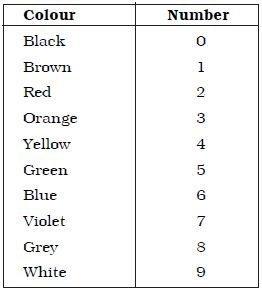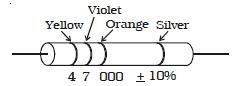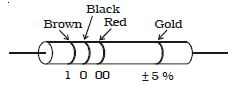Physics

# Define and Describe on Carbon Resistors

The wire wound resistors are expensive and huge in size. Hence, carbon resistors are used. Carbon resistor consists of a ceramic core, on which a thin layer of crystalline carbon is deposited. These resistors are cheaper, stable and small in size. The resistance of a carbon resistor is indicated by the colour code drawn on it (Table).

Tab: Colour code for carbon resistorsA three colour code carbon resistor is discussed here. The silver or gold ring at one end corresponds to the tolerance. It is a tolerable range ( ± ) of the resistance. The tolerance of silver, gold, red and brown rings is 10%, 5%, 2% and 1% respectively. If there is no coloured ring at this end, the tolerance is 20%. The first two rings at the other end of tolerance ring are significant figures of resistance in ohm. The third ring indicates the powers of 10 to be multiplied or number of zeroes following the significant figure.

Example:

The first yellow ring in Figure corresponds to 4. The next violet ring corresponds to 7. The third orange ring corresponds to 103. The silver ring represents 10% tolerance. The total resistance is 47 × 103 + 10% i.e. 47 k Ω, 10%.Fig: Carbon resistor colour code

Fig 2 shows 1 k Ω, 5% carbon resistor.Presently four colour code carbon resistors are also used. For certain critical applications 1% and 2% tolerance resistors are used.Fig: Carbon resistor